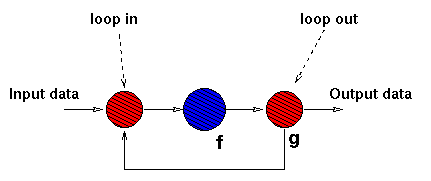In a loop, each data item x appearing onto the output stream is processed by a function f. The result is processed by using another (boolean function) g. If the result of g(f x) is true, then (f x) is submitted again to the loop input, otherwise, (f x) is delivered onto the output channel.

Functionally a loop has type

'a stream -> 'a stream
provided that the functions f and g have type

'a -> 'a

'a -> bool

Given the input stream :xn:...:x1:x0: the loop loop(c,f) computes the output stream :f(f(f ...(f xn) ...)):...:f(f ...(f x0) ...), where the number of times f is computed onto each stream item depends on the number of times the function c computed onto the same data holds true. In other words each element of the output data stream evaluates to: let rec loop c f x = if (c (f x)) then (loop c f (f x)) else (f x) in (loop c f (xi))

In terms of (parallel) processes, a sequence of data appearing onto the input stream of a loop is submitted to a loop in stage. This stage just merges data coming from the input channel and from the feedback channel and delivers them to the loop body stage. The loop body stage computes f and delivers results to the loop end stage. This latter stage computes g and either delivers (f x) onto the output channel or it delivers the value to the loop in process along the feedback channel. The resulting process network looks like the following:Have a look at the loop behaviour, in terms of data items processed.

Back to the skeleton set page.